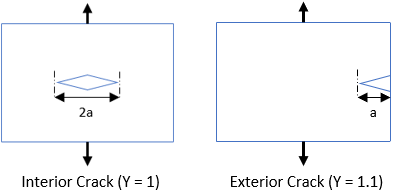## Fracture Toughness

Consider a part is formed by bending a sheet of 52100 Steel, which has a Fracture Toughness of 14.3 MPa∙m^(1/2), and a yield strength of 586 MPa. What is the total max crack length that can be tolerated for an interior crack in mm?

Hint
$$K_{IC}=Y\cdot \sigma \cdot \sqrt{\pi (a)}$$$where $$K_{IC}$$ is fracture toughness, $$\sigma$$ is applied stress, $$a$$ is crack length, and $$Y$$ is geometrical factor. Hint 2Fracture toughness is the stress intensity of when a brittle material will fail due to the combination of an applied stress and crack length. $$K_{IC}=Y\cdot \sigma \cdot \sqrt{\pi (a)}$$$
where $$K_{IC}$$ is fracture toughness, $$\sigma$$ is applied stress, $$a$$ is crack length, and $$Y$$ is geometrical factor.Based on the problem statement:
• $$\sigma=586\:MPa$$
• $$Y=1$$ (since an interior crack will be produced)
• $$K_{IC}=14.3\:MPa\cdot m^{1/2}$$
Solving for crack length:
$$a=\left (\frac{K_{IC}}{Y\cdot \sigma} \right )^2\cdot \frac{1}{\pi}$$$$$a=\left (\frac{14.3MPa\cdot \sqrt{m}}{(1)(586MPa)} \right )^2\cdot \frac{1}{\pi}=\frac{0.000595m}{\pi}=0.0001896m=0.1896\:mm$$$
Because an interior crack has a total crack length of $$2 \times a$$ (based on the figure):
$$0.1896mm\times 2=0.38\:mm$$\$
0.38 mm